Intermediate Algebra 2e

# Practice Test

### Practice Test

537.

Plot each point in a rectangular coordinate system.

$(2,5)(2,5)$
$(−1,−3)(−1,−3)$
$(0,2)(0,2)$
$(−4,32)(−4,32)$
$(5,0)(5,0)$

538.

Which of the given ordered pairs are solutions to the equation $3x−y=6?3x−y=6?$

$(3,3)(3,3)$ $(2,0)(2,0)$ $(4,−6)(4,−6)$

Find the slope of each line shown.

539.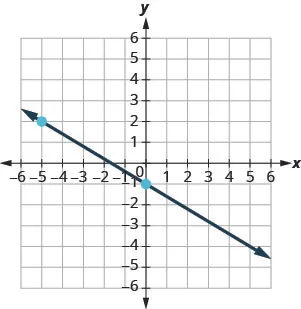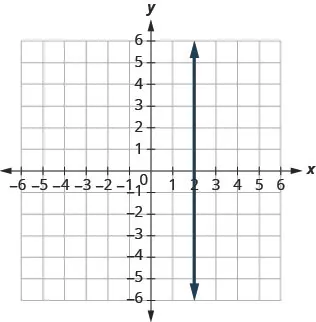540.

Find the slope of the line between the points $(5,2)(5,2)$ and $(−1,−4).(−1,−4).$

541.

Graph the line with slope $1212$ containing the point $(−3,−4).(−3,−4).$

542.

Find the intercepts of $4x+2y=−84x+2y=−8$ and graph.

Graph the line for each of the following equations.

543.

$y = 5 3 x − 1 y = 5 3 x − 1$

544.

$y = − x y = − x$

545.

$y = 2 y = 2$

Find the equation of each line. Write the equation in slope-intercept form.

546.

slope $−34−34$ and $yy$-intercept $(0,−2)(0,−2)$

547.

$m=2,m=2,$ point $(−3,−1)(−3,−1)$

548.

containing $(10,1)(10,1)$ and $(6,−1)(6,−1)$

549.

perpendicular to the line $y=54x+2,y=54x+2,$ containing the point $(−10,3)(−10,3)$

550.

Write the inequality shown by the graph with the boundary line $y=−x−3.y=−x−3.$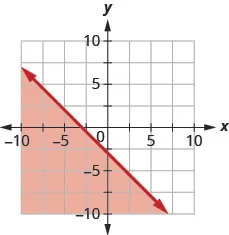Graph each linear inequality.

551.

$y > 3 2 x + 5 y > 3 2 x + 5$

552.

$x − y ≥ −4 x − y ≥ −4$

553.

$y ≤ −5 x y ≤ −5 x$

554.

Hiro works two part time jobs in order to earn enough money to meet her obligations of at least $450 a week. Her job at the mall pays$10 an hour and her administrative assistant job on campus pays $15 an hour. How many hours does Hiro need to work at each job to earn at least$450?

Let x be the number of hours she works at the mall and let y be the number of hours she works as administrative assistant. Write an inequality that would model this situation.
Graph the inequality .
Find three ordered pairs$(x,y)(x,y)$ that would be solutions to the inequality. Then explain what that means for Hiro.

555.

Use the set of ordered pairs to determine whether the relation is a function, find the domain of the relation, and find the range of the relation.

${ ( −3 , 27 ) , ( −2 , 8 ) , ( −1 , 1 ) , ( 0 , 0 ) , { ( −3 , 27 ) , ( −2 , 8 ) , ( −1 , 1 ) , ( 0 , 0 ) ,$
$( 1 , 1 ) , ( 2 , 8 ) , ( 3 , 27 ) } ( 1 , 1 ) , ( 2 , 8 ) , ( 3 , 27 ) }$

556.

Evaluate the function: $f(−1)f(−1)$ $f(2)f(2)$ $f(c).f(c).$

$f ( x ) = 4 x 2 − 2 x − 3 f ( x ) = 4 x 2 − 2 x − 3$

557.

For $h(y)=3|y−1|−3,h(y)=3|y−1|−3,$ evaluate $h(−4).h(−4).$

558.

Determine whether the graph is the graph of a function. Explain your answer.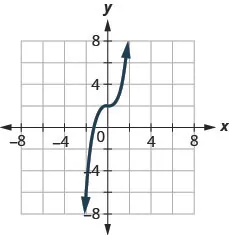In the following exercises, graph each function state its domain and range.
Write the domain and range in interval notation.

559.

$f ( x ) = x 2 + 1 f ( x ) = x 2 + 1$

560.

$f ( x ) = x + 1 f ( x ) = x + 1$

561.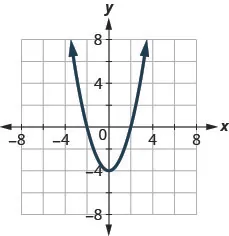Find the $xx$-intercepts.
Find the $yy$-intercepts.
Find $f(−1).f(−1).$
Find $f(1).f(1).$
Find the domain. Write it in interval notation.
Find the range. Write it in interval notation.

Order a print copy

As an Amazon Associate we earn from qualifying purchases.